## Use percentage (%) key to easily calculate amount after tip or discount

Using the percentage (%) key on Calculator, you can easily find the amount including tip or after discount. While some, like working adults, may already know how to do the percentage math with the key, many people may not know the function since they seldom use a calculator in their daily life (I actually did not know the function, though I could imagine calculation would go smoothly if I used the operation key).

### If you want to leave a 15% tip, for example, on a service that cost \$100:

With your Calculator, use the following formula to calculate the total amount including the tip.

100 + 15 % =

On the other hand, if you want to know how much the price will be after 15% discount, type this formula that gives the amount of discount applied.

100 - 15 % =

### How to calculate percentages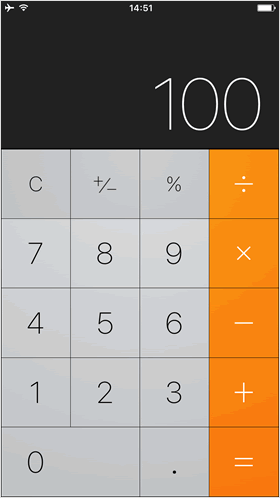To begin, enter "100" on your Calculator.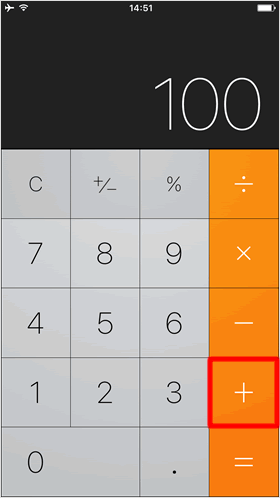Then, press the "＋" key.

* Press the "-" key, if you want to calculate the price after discount.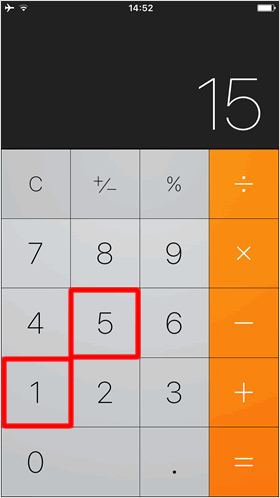Enter a percentage of the tip that you wish to leave (for example, enter "15" for 15%).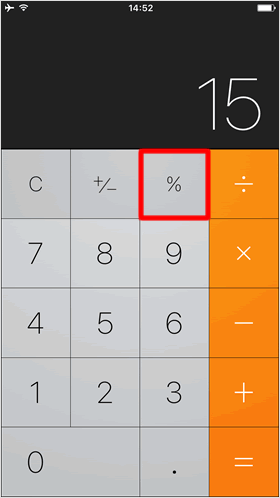Press the "%" key to show the amount of the tip on Calculator.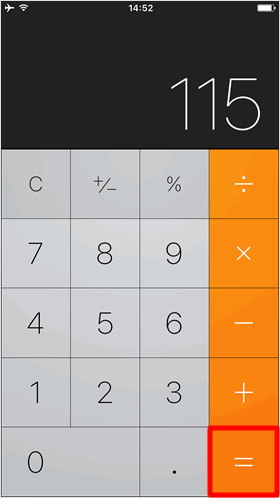By pressing the "=" key, the total amount including the tip will be displayed on the screen.